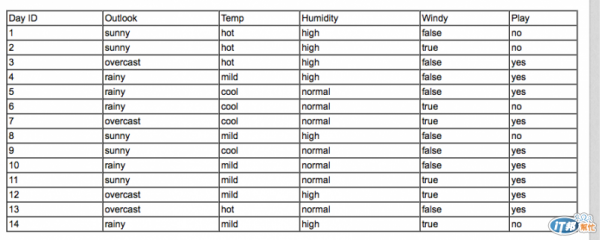DAY 14
0

[決策樹圖]

# 決定分支依據outlook(sunny=[2,3], overcast=[4,0], rainy=[3,2])

1. 計算各分支 info
info(sunny) = info([2,3]) = 0.971 bits （information 用 bits 做單位）
info(overcast) = info([4,0]) = 0.0 bits
info(rainy) = info([3,2]) = 0.971 bits

2. 算出分支總 info
info(sunny, overcast, rainy)
= info([2,3], [4,0], [3,2])
= 5/14 * 0.971 + 4/14 * 0 + 5/14 * 0.971
= 0.693 bits

3. 算出主幹 info
info(outlook) = info([9,5]) = 0.940 bits

4. 算出主幹屬性分數 gain
gain(outlook)
= info(outlook) - info(sunny, overcast, rainy)
= info([9,5]) - info([2,3], [4,0], [3,2])
= 0.940 - 0.693
= 0.247 bits

gain(outlook) = 0.247 bits 我們這邊就簡單看成以 outlook 做分支時，它所含的資訊量，數值越高代表資訊含量越多（詳細的涵義請自行 google ...）。

gain(temperature) = 0.029 bits
gain(humidity) = 0.152 bits
gain(windy) = 0.048 bits

(to be continued...)